# NCERT SOLUTIONS FOR CLASS 9 SCIENCE CHAPTER 12 SOUND

The National Council of Educational Research and Training (NCERT) is an autonomous body of the Indian government that formulates the curricula for schools in India that are governed by the Central Board of Secondary Education (CBSE) and certain state boards. Therefore, students who will be taking the Class 10 tests administered by various boards should consult this NCERT Syllabus in order to prepare for those examinations, which in turn will assist those students get a passing score.

When working through the exercises in the NCERT textbook, if you run into any type of difficulty or uncertainty, you may use the swc NCERT Solutions for class 9 as a point of reference. While you are reading the theory form textbook, it is imperative that you always have notes prepared. You should make an effort to understand things from the very beginning so that you may create a solid foundation in the topic. Use the NCERT as your parent book to ensure that you have a strong foundation. After you have finished reading the theoretical section of the textbook, you should go to additional reference books.

## NCERT SOLUTIONS FOR CLASS 9 SCIENCE CHAPTER 12 SOUND – Exercises

Question 1. How does the sound produced by a vibrating object in a medium reach your ear?
Solution : When an object vibrates, it forces the neighbouring particles of the medium to vibrate. These vibrating particles then force the particles adjacent to them to vibrate. In this way, vibrations produced by an object are transferred from one particle to another till it reaches the ear.

Question 2. Explain how sound is produced by your school bell.
Solution : When the school bell vibrates, it forces the adjacent particles in air to vibrate. This disturbance gives rise to a wave and when the bell moves forward, it pushes the air in front of it. This creates a region of high pressures known as compression. When the bell moves backwards, it creates a region of low pressure know as rarefaction. As the bell continues to move forward and backward, it produces a series of compressions and rarefactions. This makes the sound of a bell propagate through air.

Question 3. Why are sound waves called mechanical waves?
Solution : Sound waves force the medium particles to vibrate. Hence, these waves are known as mechanical waves. Sound waves propagate through a medium because of the interaction of the particles present in that medium.

Question 4. Suppose you and your friend are on the moon. Will you be able to hear any sound produced by your friend?
Solution : Sound needs a medium to propagate. Since the moon is devoid of any atmosphere, you cannot hear any sound on the moon.

Question 5. Which wave property determines (a) loudness, (b) pitch?
Solution :
(a) Amplitude (b) Frequency
(a) The loudness of a sound depends on its amplitude. If the amplitude of a sound is large, then the sound produced will also be loud.
(b) The pitch of a sound depends on its frequency. A sound will be considered a high pitched sound, if its frequency is high.

Question 6. Guess which sound has a higher pitch: guitar or car horn?
Solution : Sound of Guitar has a higher pitch.

Question 7. What are wavelength, frequency, time period and amplitude of a sound wave?
Solution : wavelength: For a sound wave, the combined length of a compression and an adjacent rarefaction is called its wavelength even the distance between centres of two consecutive compressions or two consecutive rarefactions is also equal to its wavelength.

frequency: The number of vibrations or oscillations per second is called frequency i.e. it is the number of complete waves or cycles produced in one second.

time period: The time taken to complete one vibration/oscillation/complete wave is called time period. It is measured in seconds.

amplitude: It is the maximum displacement of the particles of the medium from their mean/original position at rest.

Question 8. How are the wavelength and frequency of a sound wave related to its speed?
Solution :
From the equation: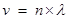where v = velocity/speed

n = frequency of wave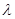= wavelength of wave

Question 9. Calculate the wavelength of a sound wave whose frequency is 220 Hz and speed is 440 m/s in a given medium.
Solution :

Since we know= 440/220 = 2 m

Question 10. A person is listening to a tone of 500 Hz sitting at a distance of 450 m from the source of the sound. What is the time interval between successive compressions from the source?
Solution :
The time interval between successive compressions from the source

T = 1/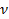= 1/500 = 0.002 second.

Question 11. Distinguish between loudness and intensity of sound.
Solution :

Question 12. In which of the three media, air, water or iron, does sound travel the fastest at a particular temperature?
Solution : Sound will travel the fastest in iron at a particular temperature.

Question 13. An echo returned in 3 s. What is the distance of the reflecting surface from the source, given that the speed of sound is 342 m s-1 ?
Solution :
Speed of sound = distance/time

therefore, distance travelled by sound during echo =Speed x time = 342 x 3 = 1026 m

so the distance of reflecting surface = 1026/2 =513 m

Question 14. Why are the ceilings of concert halls curved?
Solution : The ceilings of concert halls are curved because such architecture helps the sound to reach all the corners and places of concert hall.

Question 15. What is the audible range of the average human ear?
Solution : 20 Hz to 20,000Hz.

Question 16. What is the range of frequencies associated with
(a) Infrasound?
(b) Ultrasound?
Solution :
Infrasound = less than 20 Hz

Ultrasound = greater than 20 KHz

Question 17. A submarine emits a sonar pulse, which returns from an underwater cliff in 1.02 s. If the speed of sound in salt water is 1531 m/s, how far away is the cliff?
Solution :

Distance travelled by a sonar pulse = speed of sound in salt water x time

= 1531 x 1.02 = 1561.62 m

therefore, the distance of cliff from submarine = 1561.62/2 = 780.81 m

Question 18. What is sound and how is it produced?
Solution :
Sound is a form of energy that produces a sensation of hearing in our ears.

Sound gets produced when any object vibrates/oscillates.

Question 19. Describe with the help of a diagram, how compressions and rarefactions are produced in air near a source of sound.
Solution :

Question 20. Cite an experiment to show that sound needs a material medium for its propagation.
Solution : Take an electric bell and an airtight glass bell jar. The electric bell is suspended inside the airtight bell jar. The bell jar is connected to a vacuum pump If you press the switch you will be able to hear the bell. Now start the vacuum pump. When the air in the jar is pumped out gradually, the sound becomes fainter, although the same current is passing through the bell. After some time when less air is left inside the bell jar you will hear a very feeble sound. Now if we evacuate the bell jar no sound is heard.

Result: The above mentioned activity shows that sound needs a medium to propagate.

Question 21. Why is sound wave called a longitudinal wave?
Solution : Sound wave is called a longitudinal wave because sound waves travel in the air through compressions and rarefactions.

Question 22. Which characteristic of the sound helps you to identify your friend by his voice while sitting with others in a dark room?
Solution : Pitch of the sound wave.

Question 23. Flash and thunder are produced simultaneously. But thunder is heard a few seconds after the flash is seen, why?
Solution :

Since speed of thunder (sound) is much less (332 m/s) as compared to speed of flash (light) which is about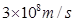therefore light travels faster than sound hence thunder is heard a few seconds after the flash is seen.

Question 24. A person has a hearing range from 20 Hz to 20 kHz. What are the typical wavelengths of sound waves in air corresponding to these two frequencies? Take the speed of sound in air as 344 m s-1 .
Solution :
For 20 Hz sound waves the wavelength would be= v/n = 344/20 = 17.2 m

For 20 kHz sound waves the wavelength would be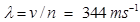/20000 Hz = 0.0172 m

Question 25. Two children are at opposite ends of an aluminium rod. One strikes the end of the rod with a stone. Find the ratio of times taken by the sound wave in air and in aluminium to reach the second child.
Solution :
Since speed of sound in air = 344 m/s

and speed of sound in aluminium = 6420 m/s

we know that v = distance/time therefore time = d/v

time taken by sound wave in air/time taken by sound wave in aluminium

= d/344: d/6420 = 6420/344 = 18.66/1

the sound will take 18.66 times more time through air than in aluminium in reaching other boy.

Question 26. The frequency of a source of sound is 100 Hz. How many times does it vibrate in a minute?
Solution :
Frequency of source of sound being 100 Hz means the sound source vibrates 100 times in one second.

therefore vibrations made by sound source in 1 min (60 sec) = 100 x 60 = 6000

Question 27. Does sound follow the same laws of reflection as light does? Explain.
Solution : Yes. Sound follows the same laws of reflection as light does. We can say that because here the directions in which the sound is incident and is reflected make equal angles with the normal to the reflecting surface at the point of incidence, and the three are in the same plane.

Question 28. When a sound is reflected from a distant object, an echo is produced. Let the distance between the reflecting surface and the source of sound production remains the same. Do you hear echo sound on a hotter day?
Solution :
As the sensation of sound persists in our brain for about 0.1 s.To hear a distinct echo the time interval between the original sound and the reflected one must be at least 0.1s. There for the total distance covered by the sound from the point of generation to the reflecting surface and back should be at least  (344 m/s) x 0.1 s= 34.4 m. Thus, for hearing distinct echoes, the minimum distance of the obstacle from the source of sound must be half of this distance, that is, 17.2 m. Speed of sound will increase with increase in temperature. Therefore, on a hotter day speed of sound will be greater hence echoes may be heard more than once because of multiple reflections of sound result will be no distinct echo will be heard by us.

Question 29. Give two practical applications of reflection of sound waves.
Solution :
Two practical applications of reflection of sound waves

(i) Megaphones or loudhailers, horns, musical instruments such as trumpets and shehanais, are all designed to send sound in a particular direction without spreading it in all directions.

(ii) Stethoscope is a medical instrument used for listening to sounds produced within the body, chiefly in the heart or lungs. In stethoscopes the sound of the patient’s heartbeat reaches the doctor’s ears by multiple reflection of sound.

Question 30. A stone is dropped from the top of a tower 500 m high into a pond of water at the base of the tower. When is the splash heard at the top? Given, g = 10 m s-2 and speed of sound =340 m s-1 .
Solution :

v2 = u2 + 2gh

= 0 + 2 x 10 x 500

= 10000

v = 10000= 100 m/s

we also know that v = u + gt = 0 + 10t

100 = 10t or, Time taken by stone to reach the pond surface (t) = 100/10 = 10 sec

therefore, time taken by sound to reach the top from pond surface = d/v = 500/340

= 1.47 sec

so the total time taken for splash being heard at the top = 10 + 1.47 = 11.47 s

Question 31. A sound waves travels at a speed of 339 m s-1. If its wavelength is 1.5 cm, what is the frequency of the wave? Will it be audible?
Solution :

Since we know that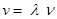339 = 0.015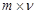ν = 339/0.015 = 22600 Hz

Since the resulting frequency is beyond the audible range of human beings (20Hz to 20kHz) therefore sound will not be audible to human ears.

Question 32. What is reverberation? How can it be reduced?
Solution : The repeated reflection of sound due to which sound persists for a long time is called reverberation. To reduce reverberation, the roof and walls of the auditorium are generally covered with sound-absorbent materials like compressed fibreboard, rough plaster or draperies. The seat materials are also selected on the basis of their sound absorbing properties.

Question 33. What is loudness of sound? What factors does it depend on?
Solution : Loudness is a measure of the response of the ear to the sound. Even when two sounds are of equal intensity, we may hear one as louder than the other simply because our ear detects it better. Loudness of sound depends upon the amplitude of those sound waves. Higher is the amplitude of vibrating air particles louder will be the sound.

Question 34. Explain how bats use ultrasound to catch a prey.
Solution : Bats search out prey and fly in dark night by emitting and detecting reflections of ultrasonic waves. The high-pitched ultrasonic squeaks of the bat are reflected from the obstacles or prey and returned to bat’s ear. The nature of reflections tells the bat where the obstacle or prey is and what it is like.

Question 35. How is ultrasound used for cleaning?
Solution : Ultrasound is generally used to clean parts located in hard-to-reach places, for example, spiral tube, odd shaped parts, electronic components etc. Objects to be cleaned are placed in a cleaning solution and ultrasonic waves are sent into the solution. Due to the high frequency, the particles of dust, grease and dirt get detached and drop out. The objects thus get thoroughly cleaned.

Question 36. Explain the working and application of a sonar.
Solution : Sonar is a device that uses ultrasonic waves to measure the distance, direction and speed of underwater objects. Sonar consists of a transmitter and a detector and is installed in a boat or a ship. The transmitter produces and transmits ultrasonic waves. These waves travel through water and after striking the object on the seabed, get reflected back and are sensed by the detector. The detector converts the ultrasonic waves into electrical signals which are appropriately interpreted. The distance of the object that reflected the sound wave can be calculated by knowing the speed of sound in water and the time interval between transmission and reception of the ultrasound. Let the time interval between transmission and reception of ultrasound signal be t and the speed of sound through seawater be v. The total distance, 2d travelled by the ultrasound is then, 2d= v x t.
The above method is called echo-ranging. The sonar technique is used to determine the depth of the sea and to locate underwater hills, valleys, submarine, icebergs, sunken ship etc.

Question 37. A sonar device on a submarine sends out a signal and receives an echo 5 s later. Calculate the speed of sound in water if the distance of the object from the submarine is 3625 m.
Solution :
Distance of object from submarine = 3625 m

therefore, distance travelled by sonar waves = 2 x 3625 = 7250 m

since, speed = distance/time= 7250/5 = 1450 m/s

Question 38. Explain how defects in a metal block can be detected using ultrasound.
Solution : Ultrasounds can be used to detect cracks and flaws in metal blocks. Metallic components are generally used in construction of big structures like buildings, bridges, machines and also scientific equipment. The cracks or holes inside the metal blocks, which are invisible from outside reduces the strength of the structure. Ultrasonic waves are allowed to pass through the metal block and detectors are used to detect the transmitted waves. If there is even a small defect, the ultrasound gets reflected back indicating the presence of the flaw or defect

Question 39. Explain how the human ear works.
Solution : Different sounds produced in our surroundings are collected by pinna that sends these sounds to the ear drum via the ear canal. The ear drum starts vibrating back and forth rapidly when the sound waves fall on it. The vibrating eardrum sets the small bone hammer into vibration. The vibrations are passed from the hammer to the second bone anvil, and finally to the third bone stirrup. The vibrating stirrup strikes on the membrane of the oval window and passes its vibration to the liquid in the cochlea. This produces electrical impulses in nerve cells. The auditory nerve carries these electrical impulses to the brain. These electrical impulses are interpreted by the brain as sound and we get a sensation of hearing.

## Conclusions for NCERT SOLUTIONS FOR CLASS 9 SCIENCE CHAPTER 12 SOUND

SWC academic staff has developed NCERT answers for this chapter of the ninth grade science curriculum. We have solutions prepared for all the ncert questions of this chapter. The answers, broken down into steps, to all of the questions included in the NCERT textbook’s chapter are provided here. Read this chapter on theory. Be certain that you have read the theory section of this chapter of the NCERT textbook and that you have learnt the formulas for the chapter that you are studying.